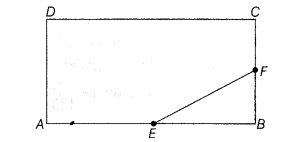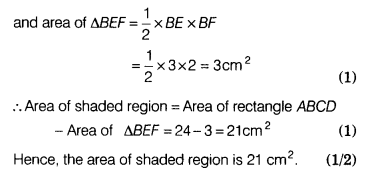# In the given figure, ABCD is a rectangle of dimension 4 cm and 6 cm

In the given figure, ABCD is a rectangle of dimension 4 cm and 6 cm. E and F are the mid-points of AB and BC, respectively. Find the area of the shaded portion.Given, AB = 6cm and BC = 4cm

Since, E and F are the mid-points of AB and BC, respectively.

∴ AE = EB = 3 cm and CF = FB = 2 cm